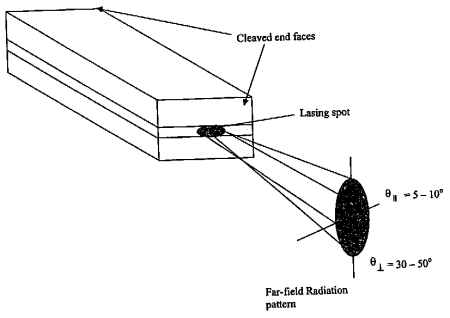# Laser Diode Characteristics

Laser Diodes (LD) are very commonly used in fiber optic communication systems and their characteristics are of primary importance. So here we give a summary of LD’s characteristics.

### Laser Diode Threshold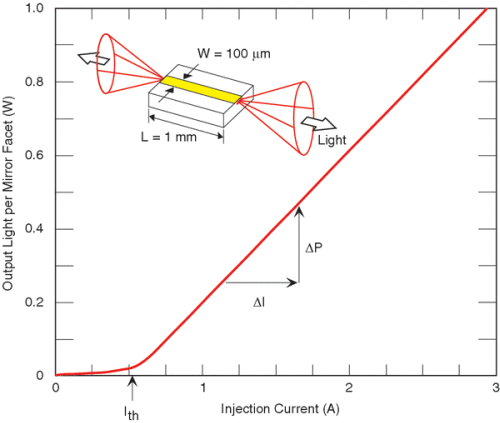The above figure shows a laser diode’s output optical power versus injected electrical current  – P/I Curve.

As we can see, the output optical power varies as a function of the current passing through the diode. When biased below a threshold current Ith, the output power is very low. But as the current increases over the threshold Ith, the output optical power increases significantly at a sharp slope. Threshold currents lie typically in the range of 25 – 250 mA and the output powers are typically in the range of 1 – 10 mW.

> Slope Efficiency

The P/I curve slope above threshold current is called a LD’s slope efficiency and is defined as dP/dI. Here dP is the change in the light power output, and dI is the change in the forward current through the diode. Slope efficiency has a unit of mW/mA.

> Differential external quantum efficiency

The increase in the number of electrons injected per unit time into the laser is dI/e, where e is the electronic charge. The additional number of photons exiting the laser is dP/hv, where v is the frequency of the radiation and h is the Planck constant. Differential external quantum efficiency of the laser ηD is defined as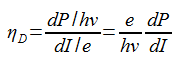ηD has a value between 0.25 and 0.6 for continuous wave lasers.

> Temperature Dependence of Laser Diode Threshold and Output Power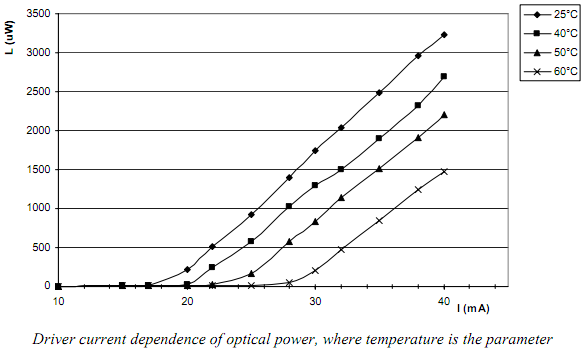Laser diodes’ threshold and output power have a strong dependence on temperature. The above figure shows the P/I curve at different temperatures.

We can see that the threshold drifts considerably following the temperature change. The relationship between LD’s threshold current and the temperature is calculated by the following formula.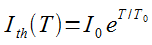where I0 is a constant and T0 is known as the characteristic temperature of the diode. Typically, Ith is about 0.6-1% per °C for GaAlAS lasers.

### Laser Diode Output Spectrum

> Input Current Under Threshold

When LDs are under threshold current, they behave like a LED and the output light come from spontaneous emission. As a result, the output wavelength spectrum is very broad just as LEDs do. The following figure shows the output spectrum of a laser diode when injected current is under its threshold.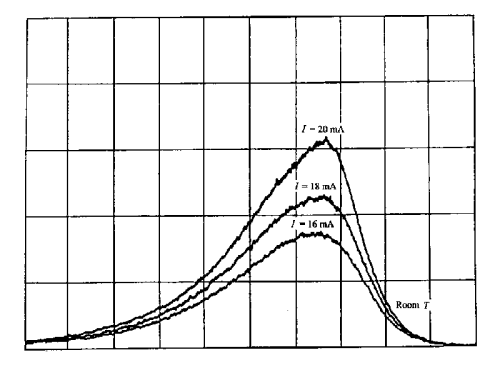> Input Current Above Threshold

As the input current is increased and above threshold, the frequencies having a larger gain and smaller cavity loss begin to oscillate and the output spectrum changes significantly. The output power also increases significantly. The following figure shows the output spectrum of a laser diode above threshold current.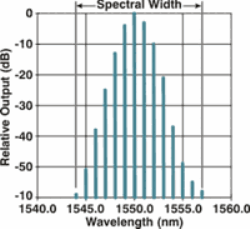In a multilongitudinal laser, the output spectrum consists of a series of wavelengths (as the peaks shown in above figure).

> Multilongitudinal Laser Diode

Each wavelength in the figure is called a longitudinal mode. When the frequency spacing Δv between adjacent modes is far less than the frequency v itself,  Δv can be calculated with the following equation.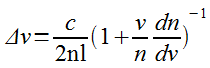where n is the refractive index of the semiconductor material, l is the cavity length.

For a typical design of n = 3.6, l = 250um,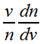= 0.38, we get a Δv = 125 GHz. For wavelength λ = 850nm, the corresponding wavelength spacing is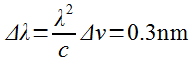> Single Longitudinal Mode Laser Diode

Multilongitudinal lasers have a significant disadvantage in fiber optic communication system – they lead to larger pulse dispersion. (You can refer to this optical fiber dispersion tutorial). So in practical fiber optic systems, we almost always use single longitudinal mode laser.

In single longitudinal laser, some components or mechanisms are built into the laser cavity that result in a loss for all longitudinal modes except one. Only this low loss longitudinal mode can oscillate. The following figure shows this type of laser’s spectrum.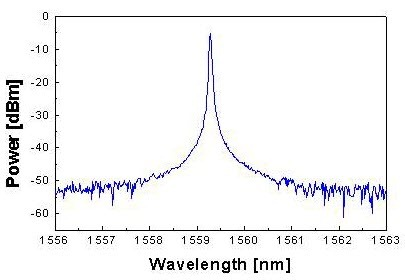> DFB Laser

A distributed feedback laser (DFB) is the most popular single longitudinal mode laser as we introduced above. In a DFB laser, the active region of the device is periodically structured as a diffraction grating. The structure builds a one dimensional interference grating and the grating provides  optical feedback for the laser.

DFB laser diodes do not use two discrete mirrors to form the optical cavity as in conventional laser design. The grating acts as the wavelength selective element for at least one of the mirrors and provides feedback, reflecting light back into the cavity to form the resonator. The grating is constructed so as to reflect only a narrow band of wavelengths, and thus produce a single longitudinal lasing mode.

You can see the structure and output spectrum of a DFB laser in the following figure.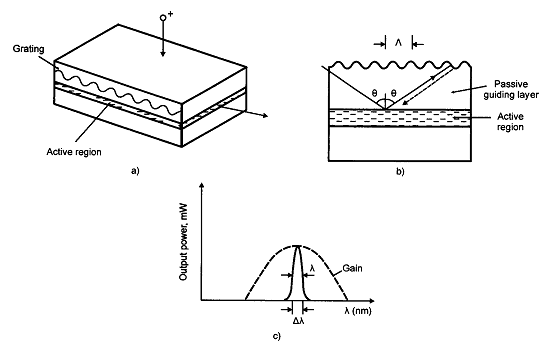### Laser Diode Mode Field Distribution

In a laser structure, the optical radiation is confined in both the lateral and transverse directions by an index step. The guiding region acts like an optical waveguide, and an optical waveguide is characterized by various transverse modes of propagation.

Most laser structures for optical fiber communication operate under a single transverse mode condition. We can approximate the mode field distribution by a Gaussian function with two different widths along the transverse (wT) and lateral (wL) direction, as shown below.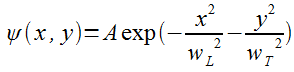where x and y represent axes parallel and perpendicular to the junction plane.

The corresponding far-field pattern will be elliptical with a larger divergence in a plane perpendicular to the junction plane. The divergences parallel and perpendicular to the junction plane are typically 5-10° and 30-50° respectively.